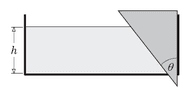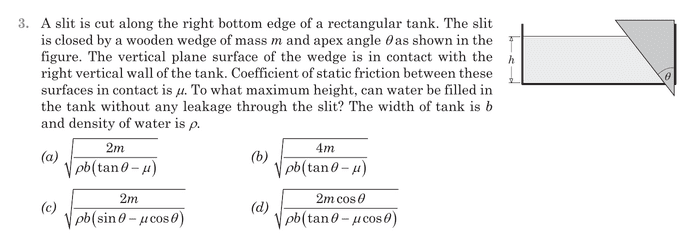# Doubt related to buoyant force and fluid thrust force

Hamiltonian
Homework Statement:
Mark all the forces acting on the wedge (the coefficient of friction between the wall and the wedge is mu) the container is of depth h and breadth b.
The wedge is placed in a fluid of density d.
Relevant Equations:
-(this problem is slightly modified from the original problem.)
There is a contact force(friction and normal force) between the wedge and the walls of the container and there is a fluid thrust acting on the side of the wedge in contact with the fluid( this force is normal to the slanted surface and the force per depth can be given by the are of the pressure diagram) there is also a buoyant force acting on this wedge in the upward direction.
$$F_{thrust} = \frac {dgh^2 b}{2cos\theta}$$
$$F_{buoyant} = dg((1/2) h^2 b tan\theta)$$
$$f = \frac{\mu dgh^2 b}{2}$$
$$F_{g} = mg$$

The normal reaction on the wedge is the horizontal component of ##F_{thrust}## and my confusion is, shouldn't ##F_{buoyant} ## actually not be a separate force rather it should be the vertical component of the force ##F_{thrust}##?
isn't the buoyant force a result of all the ##(da)P## (where ##da## is the differential area and P is the pressure at that point in the liquid)
If that's not the case what exactly is causing the buoyant force?

Last edited:

Homework Helper
Gold Member
2022 Award
The buoyancy force is the vertical component of the normal force the water exerts on the wedge.
I am confused by this: "The normal reaction on the wedge is the horizontal component of Fthrust". Which normal reaction, from water or wall?

Hamiltonian
"The normal reaction on the wedge is the horizontal component of Fthrust". Which normal reaction, from water or wall?

( the normal reaction from the water is ##F_{thrust}##)
the x-component of ##F_{thrust}## is equal to the normal reaction form the wall.

Homework Helper
Gold Member
2022 Award
( the normal reaction from the water is ##F_{thrust}##)
the x-component of ##F_{thrust}## is equal to the normal reaction form the wall.
Ok, but why doesn't the angle feature in your expression for the frictional force?

Hamiltonian
The buoyancy force is the vertical component of the normal force the water exerts on the wedge.
but in this question, there is supposed to be another force ##F_{buoyant} = (1/2)dgh^2b tan\theta## supposed to be acting on the wedge which is in addition to the y component of ##F_{thrust}##

Homework Helper
Gold Member
2022 Award
but in this question, there is supposed to be another force ##F_{buoyant} = (1/2)dgh^2b tan\theta## supposed to be acting on the wedge which is in addition to the y component of ##F_{thrust}##
It will help if you clarify which parts of your post are from the source text and which are your own thoughts.

Hamiltonianthis is the original question.
option (a) is the correct answer.

Which can be obtained by balancing all the forces on the wedge.
In doing so the problem I faced was is whether the buoyant force acting on this wedge the Y-component of ##F_{thrust}## or whether the buoyant force is ##F_{buoyant} = (1/2)dgh^2b tan\theta## (given by Archimedes principle) and if the latter is correct what is exactly causing this force to act?

Hamiltonian
Ok, but why doesn't the angle feature in your expression for the frictional force?
It gets cancelled.
$$f=\mu F_{thrust} cos\theta$$
$$F_{thrust} = \frac {dgh^2 b}{2cos\theta}$$

Homework Helper
Gold Member
2022 Award
It gets cancelled.
$$f=\mu F_{thrust} cos\theta$$
$$F_{thrust} = \frac {dgh^2 b}{2cos\theta}$$
So it does.

The y component of Fthrust is ##F_{thrust}\sin(\theta)=\frac {dgh^2 b\tan(\theta)}{2}##, as you calculated for the buoyant force. They're the same thing.

•Hamiltonian
Hamiltonian
Thanks!

I am going to go now